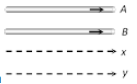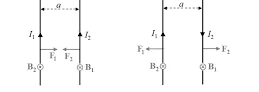#### A and B are two conductors carrying a current i in the same direction. x and y are two electron beams moving in the same directionOption 1) There will be repulsion between A and B attraction between x and y   Option 2) There will be attraction between A and B, repulsion between x and y Option 3) There will be repulsion between A and B and also x and y   Option 4) There will be attraction between A and B and also x and yAs we learnt in

force between two parallel current carrying conductors -- whereinI1 and I2 current carrying two parallel wires

a-seperation between two wires

Two parallel current attract each other and hence A and B will attract each other.

e- & e- will repel each other as these two are similar charges.

Option 1)

There will be repulsion between A and B attraction between x and y

incorrect

Option 2)

There will be attraction between A and B, repulsion between x and y

correct

Option 3)

There will be repulsion between A and B and also x and y

incorrect

Option 4)

There will be attraction between A and B and also x and y

incorrect